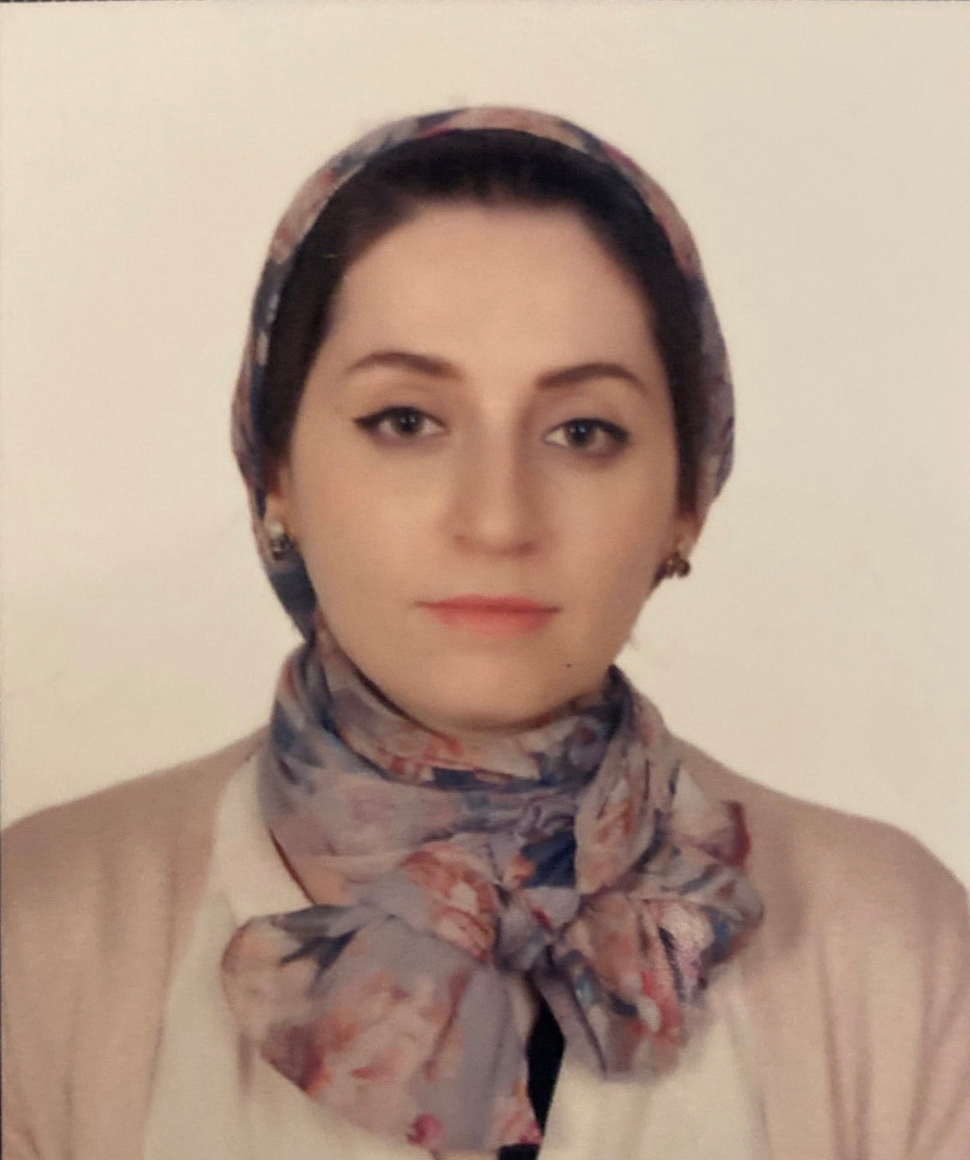# College of Science

## Theory of Computation

 Course Code CMSC312 Th 3 Pr 3 CrHrs 3

#### LecturerEman Ahmed Khorsheed Assistant Lecturer

Introduction to theory of computation, Language, Finite languages, Grammars, Derivation of grammar, Derivation Tree, Notation of Grammar, Reduced Grammar, Chomsky Normal Form), Regular expression, Finite Automata (FA), Non deterministic Finite Automata (NDFA), Deterministic Finite Automata (DFA).

• This course is divided into two parts. The first part concerned for an introductory on formal languages, automata, computability, and related matters. The objective of this part to make student able to understand grammar, derivation tree, normal form, automata and regular expression.

The second part is based on explaining the main phases of compiler. It also, make students able to understand the compiler design and write some programs (Using Visual C#) that represented basic functionalities of analysis part of compiler (Lexical and Syntax).

 Distribution of Marks Final Mark Final Exam Second Term Mid-Year First Term 100 Prac. Theor. Prac. Theor. Prac. Theor. Prac. Theor. 20 30 5 5 10 20 5 5
 References SN P. LINZ “An Introduction to Formal Languages and Automata” , 5th edition Jones & Bartlett Learning, 2012. 1 Alfred V. Aho, Monica S. Lam, Ravi Sethi, and Jeffrey D. Ullman “Compilers: Principles, Techniques, and Tools”, 2nd edition, Addison-Wesley, 2006. 2 Dick Grune, Henri E. Bal, Ceriel J. H. Jacobs, Koen G. Langendoen “ Modern  Compiler  Design”, John Wiley & Sons, 2000. 3
 Introduction to theory of computation Language Grammars Context free grammar Leftmost and Rightmost Derivations Derivation trees Simplification of Context Free Grammar Reduced grammar Chomsky Normal Form Regular Expression Regular Expression Finite Automata NFA DFA (cont.)